# Difference between revisions of "Module/guidance vector field"

## Introduction

We have written an algorithm for solving the problem of tracking smooth curves by an unmanned aerial vehicle travelling with a constant airspeed and under a wind disturbance. The algorithm is based on the idea of following a guiding vector field which is constructed from the implicit function that describes the desired (possibly time-varying) trajectory.

For fixed-wings the output of the algorithm can be directly expressed in terms of the bank angle of the UAV in order to achieve coordinated turns. Furthermore, the algorithm can be tuned offline such that physical constraints of the UAV, e.g. the maximum bank angle, will not be violated in a neighborhood of the desired trajectory.

The implementation for rotorcraft is still in the TO DO list.

This work is based on the paper Guidance algorithm for smooth trajectory tracking of a fixed wing UAV flying in wind flows, submitted to ICRA 2017 (pending of approval).

## How does the algorithm work?

We start from the implicit equation of the trajectory, for example for a circumference we have that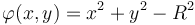$\varphi(x,y) = x^2 + y^2 - R^2$, where$x, y$ and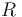$R$ are the x, y coordinates with respect to HOME (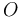$O$ in the following figure) and R is the radius of the circumference. Note that when the vehicle is over the desired trajectory, then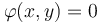$\varphi(x,y) = 0$, otherwise it will be different from zero. We will use this concept of "level set" to define the notion of distance to the trajectory, namely$e := \varphi(x,y)$. It has to be noted that this is different from the Euclidean distance (see figure below).

For the sake of clarity we will consider just 2D trajectories and parallel to the ground. Let us stack the x and y coordinates in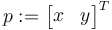$p := \begin{bmatrix}x & y\end{bmatrix}^T$ and consider the following kinematical model for our vehicle$\begin{cases} \dot p &= sm(\psi) + w \\ \dot\psi &= u, \end{cases}$

where$s\in\mathbb{R}^+$ is a constant that can be considered as the airspeed,$m = \begin{bmatrix}\cos(\psi) & \sin(\psi)\end{bmatrix}^T$ with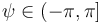$\psi\in(-\pi, \pi]$ being the attitude yaw angle,$w\in\mathbb{R}^2$ is a constant with respect to$O$ representing the wind and$u$ is the control action that will make the vehicle to turn. We also notice that the course heading$\chi\in(-\pi, \pi]$, i.e. the direction the velocity vector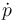$\dot p$ is pointing at, in general is different from the yaw angle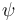$\psi$ because of the wind.

Given a desired trajectory, we compute its normal$n(p) := \nabla \varphi(p)$ and its tangent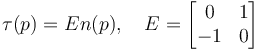$\tau(p) = En(p), \quad E=\begin{bmatrix}0 & 1 \\ -1 & 0\end{bmatrix}$. The idea is to steer the vehicle such that it follows the direction given by the unit vector calculated from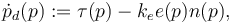$\dot p_d(p) := \tau(p) - k_e e(p)n(p),$

where$k_e$ is a positive gain. At each point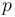$p$ we can build a unit vector from$\dot p_d(p)$. This collection of vectors is our Guidance Vector Field. Note that when the error is zero, then we are just tracking the tangent to the trajectory.

We assume that the trajectory is twice differentiable (so its gradient and Hessian exists) and that it is regular in a neighborhood of$\mathcal{P}$, i.e.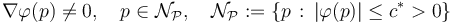$\nabla\varphi(p) \neq 0, \quad p\in \mathcal{N}_{\mathcal{P}},\quad \mathcal{N}_{\mathcal{P}} := \{p \, : \, |\varphi(p)| \leq c^* > 0\}$.

The algorithm needs for work the measurements of$\dot p$ (typically derived from GPS), position$p$ w.r.t. HOME (typically derived from GPS) and yaw angle$\psi$ or sideslip angle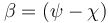$\beta = (\psi - \chi)$. The algorithm still works quite well in practice with only$\dot p$ and$p$, in other words, you will only need to have installed a GPS.

## Using the GVF in the flight plan

{TODO: to enumerate all the functions available for the user.}

So far we have added support for two kind of trajectories, ellipses and sinusoidals. Note that the former includes circumferences and the latter includes straight lines (zero frequency).

For tracking an ellipse, one has to call the function

<call fun="gvf_ellipse(WP_STDBY, gvf_ellipse_a, gvf_ellipse_b, gvf_ellipse_alpha)"/>


where gvf_ellipse_a (horizontal axis), gvf_ellipse_b (vertical axis), gvf_ellipse_alpha (rotation w.r.t. North) can be defined in your settings file.

For tracking a straight line that crosses HOME with course heading 45 degrees

<call fun="gvf_line_wp_heading(WP_HOME, 45)"/>


For tracking a sinusoidal, the same as the straight line but including the additional information about frequency, offset and amplitude (in meters)

<call fun="gvf_sin_wp_heading(WP_HOME, course_heading, freq, off_set, amplitude)"/>


For setting the tracking direction (the direction of the tangent vector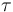$\tau$ in the above figure)

<call fun="gvf_set_direction(s)"/>


where s is an integer number 1 or -1.

## Gain tunning

The user has to tune two gains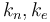$k_n, k_e$. The former one determines the convergence rate for aligning the vehicle to the vector field. A typical value for starting tuning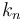$k_n$ should be between 0.2 and 1. The latter gain determines how aggressive is the vector field. For example, in the following figure we have an ellipse with two different values of$k_e$, at the left we have a value of 3 and at the right 0.4. Note how in left one the vectors are "more aggressive" towards the trajectory. While a big value can make the vehicle to converge quickly to the trajectory, it can make the tracking unstable once the vehicle is close it. This is because the vector field might change so quick that physically the vehicle cannot follow it. Check the Section IV in the original paper in the introduction.

It is a good practice in the flight plan to set the gains before calling the trajectory since it is pretty common to have different gains for different trajectories. For example

<call fun="gvf_set_direction(s)"/>
<call fun="gvf_set_gains(ke, kn)"/>
<call fun="gvf_ellipse(WP_STDBY, gvf_ellipse_a, gvf_ellipse_b, gvf_ellipse_alpha)"/>


## TO DO list

• Better integration of the GVF with the ground station
• Support to rotorcrafts